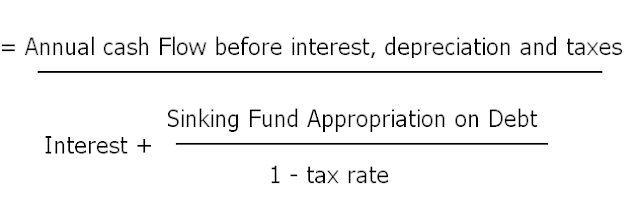Cash flow to debt service ratio is the improvement in interest coverage ratio. In interest coverage ratio, we create relationship between net profit and total interest payment but we all know that interest payment will always in cash form. So, it will be better if we create relationship between cash flow  from operation instead of net profit and total interest payment. Following is the formula of cash flow to debt service ratio.

In this formula, we are seeing that there is relationship between cash inflow from operation and total interest payment. More high will our cash flow before interest, depreciation and taxes, more we can easily pay our interest without any problem.

What is sinking fund Appropriation on Debt?

Sinking fund is just like a reserve fund which is used for repayment of loan. So, this fund is also created from our profit for repayment of loan. This fund will be specific rate on our taken debt. So, we will add this total interest payment when we calculate cash flow to debt service ratio.

Example

Calculate cash to debt service ratio :

Net profit after tax = 22500

Fixed interest charges = 2000

Depreciation charged = 3000

Tax rate = 50%

Sinking fund appropriation = 7.5% of outstanding debentures

10% debentures = 20000

Debt cash flow coverage ratio or cash flow to debt service ratio =

CF/ 1 + SFD / 1 - T

= 22500 + 22500 ( tax ) + 2000 ( interest ) + 3000 ( Depreciation ) / 2000 + 1500 / 1- 50%

= 50000 / 2000 + 1500 / 0.5

= 50000 /5000 = 10 times

It means we have 10 times cash from our profit which is available for paying interest. In other words, we have cash profit for paying interest on the debt of 200,000.

Importance of Cash Flow to Debt Service Ratio

To calculate this ratio is very important for creditor because our creditor will think 100 times before giving us loan. If he see,  we have 10 times cash profit for paying his interest on loan, he will ready to give us more loan. If this cash flow to debt service ratio will be 20 times, other creditors will also be ready to give us loan. If our cash flow to debt service is just 1 or 2 times, our creditors can say us good bye, when we will ask for loan..

## \$type=three\$a=hide\$cm=hide\$s=hide\$show=/2019/06/10-steps-to-become-better-investor.html\$l=hide

Name

false
ltr
item
Accounting Education: Cash Flow to Debt Service Ratio
Cash Flow to Debt Service Ratio
http://3.bp.blogspot.com/-0xu_-vr-LVQ/Ttx8Y6BF5hI/AAAAAAAAHIU/E5BeXvwdV9I/s640/formula.PNG
http://3.bp.blogspot.com/-0xu_-vr-LVQ/Ttx8Y6BF5hI/AAAAAAAAHIU/E5BeXvwdV9I/s72-c/formula.PNG
Accounting Education
http://www.svtuition.org/2011/12/cash-flow-to-debt-service-ratio.html
http://www.svtuition.org/
http://www.svtuition.org/
http://www.svtuition.org/2011/12/cash-flow-to-debt-service-ratio.html
true
2410664366776677676
UTF-8